## Natural Frequency and its significance for Earthquake Resistant Design

Natural frequency is a fundamental concept in physics and engineering that describes the frequency at which a system oscillates when disturbed from its equilibrium position. Although it’s an essential concept,  many of us fail when we try to communicate this terminology. In this blog, I will explain natural frequency in simple terms and provide demonstrations to help illustrate its significance. Additionally, we will discuss its importance in earthquake-resistant building design.

### Natural Frequency

The natural frequency of a system is the frequency at which it oscillates when it is disturbed from its equilibrium position with no external forces acting on it. This frequency is determined by the system’s properties, such as its mass, stiffness, and damping. The natural frequency is often denoted by the symbol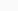(omega) and is measured in Hertz (Hz).

For example, consider a mass hanging from a spring. When the mass is pulled down and released, it oscillates up and down at a certain frequency. This frequency is the natural frequency of the system and is given by:

(1)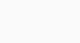where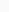is the spring constant and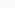is the mass.

Equation 1 provides the natural frequency in an ideal scenario where there is no damping. However, in reality, there is energy dissipation in each oscillation cycle, which is known as damping. To account for this, we need to incorporate a damping coefficient (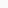) into the system. The resulting damped natural frequency is given by:

(2)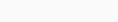where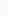is the damping ratio and its expression in terms of damping coefficient is given as:

(3)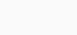whereis the damping coefficient of the system.

### Simulation of a SDOF System

Now that we have examined the fundamental equation for natural frequency, let’s explore this concept through a simulation of a single-degree-of-freedom system. To simulate the motion of a single-degree-of-freedom system with no external force, we need to solve the equation of motion. This equation can be expressed as follows:

(4)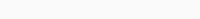where,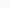is the displacement from the mean postion,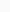is the velocity andis the acceleration.

Consider a system with massattached to a spring with stiffnessand a damping ratio of. Suppose we initially pull the system to a distance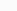from its equilibrium position and then release it. The system will then oscillate back and forth at a certain frequency, which is the natural frequency of the system.If we apply a larger displacement to the system initially, we might expect it to vibrate at a different frequency than when we apply a smaller displacement. However, this is not the case. The natural frequency remains constant, regardless of the initial displacement. The only difference between the two scenarios is the amplitude of the vibration. When we apply a larger initial displacement, the system oscillates with a greater amplitude, resulting in more rapid vibration. Conversely, when we apply a smaller initial displacement, the system oscillates with a smaller amplitude, resulting in a slower vibration. Thus, the natural frequency of the system remains constant, while the amplitude of the vibration changes with the initial displacement.

Now let’s look at the simulation for two different cases: a) undamped condition, and b) damped condition.

a.)   Undamped Case:

If we put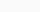in equation 4 and solve forthen we get the expression:

(5)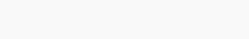whereis the initial displacement and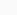is the velocity.

Based on the above equation, the result of the simulation in python is as follows:b.) Damped Case:

Considering the damping coefficient in equation 4 the solution to the equation is:

(6)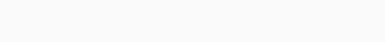The result of the simulation of this equation in python is as follows:From the simulations presented above, it is evident that when an initial displacement is applied to a single degree of freedom system and released, it oscillates in a harmonic manner. This oscillation frequency is known as the natural frequency of the system, which is determined by the system’s properties such as its mass, stiffness, and damping. The natural frequency is a fundamental characteristic of the system and plays a crucial role in determining its behavior.

### Resonance:

Since we have understood the natural frequency, we can talk about resonance. Resonance is a phenomenon that occurs when a system is forced to oscillate at its natural frequency or a multiple of its natural frequency. When a system is excited by an external force at or near its natural frequency, the amplitude of the system’s oscillation can increase dramatically, even if the external force is relatively weak. This is because the energy supplied by the external force is being added to the system at just the right time to reinforce its oscillations.

Resonance can be beneficial or detrimental, depending on the context. For example, in musical instruments, resonance is used to amplify sound by matching the frequency of the sound wave to the natural frequency of the instrument. In contrast, resonance can be a cause of structural failure in buildings and bridges, as the oscillations caused by external forces can become so large that they cause the structure to vibrate uncontrollably, leading to damage or collapse.

During an earthquake, resonance can occur in a building when the frequency of ground motion matches with the natural frequency of the building. The natural frequency of a building varies depending on its mass and stiffness as shown in the equation 1. For example, a tall building will have a lower natural frequency compared to a shorter building. Similarly, if we increase the stiffness of a building with the addition of lateral load-resisting members like shear walls, then the natural frequency increases.

In addition, there are several factors that affect the frequency content of an earthquake, such as the type of soil. If the soil is rocky, the frequency of vibration is high but the amplitude is low. Conversely, if the soil is relatively loose, the frequency of vibration is low but the amplitude is high. If the frequency of ground motion matches with the natural frequency of the building, it can cause devastating effects.

The resonance condition is clearly seen in the simulation below where three buildings with different heights are placed in a Shake Table. The Shake Table is built by me and my friends at the research lab of Khwopa College of Engineering. . In the simulation, the input is a discontinuous sine wave signal with varying frequency, starting at 5 Hz and ending at 0.8 Hz with a decrement of 0.2 Hz every 5 seconds. Similarly, the amplitude increases from 5 mm to 20 mm. The graph of input motion is shown below:Figure: Input motion of the Simulation

In the simulation, it can be observed that during the initial high frequency of the ground motion, the shortest building vibrates more compared to the other two buildings. However, as the frequency gradually decreases, the middle building starts to vibrate more. Finally, when the frequency becomes very low, the tall building vibrates significantly more than the other two buildings. This indicates that the buildings with different heights will have different natural frequencies, and they will vibrate differently in response to ground motion with varying frequencies.

### Earthquake Resistant design

From the above simulation, we can say that resonance can cause serious damage to structures during an earthquake. Therefore, it’s essential to design buildings in such a way that they do not resonate with the ground motion during an earthquake. To achieve this, we must first understand the ground motion parameters of the site where the building is to be constructed. These parameters are directly dependent on soil conditions, nearest faults, past seismic activities, etc., and are obtained through various seismic analyses. Based on the information we plot a response spectrum of the ground motion that is most likely to occur.

Based on the response spectrum, engineers design the building to have a natural frequency that is different from the ground motion. If the building’s natural frequency matches the frequency of the ground motion during an earthquake, resonance can occur, and the structure can suffer serious damage. To prevent this, engineers adjust the mass and stiffness of the building so that it does not resonate with the ground motion. This is typically achieved by adding lateral load-resisting elements like shear walls to increase the building’s stiffness, thus increasing its natural frequency. Also, other methods like tuned mass damper and base isolation can also be considered. By designing buildings in this way, we can reduce the risk of damage and ensure the safety of people and property during an earthquake.

The python code and simulation results are attached below:

Damped Oscillation – GIF

Undamped Oscillation – GIF

Damped Osciallation – Mp4

Undamped Osciallation – Mp4

Python Code – GitHub